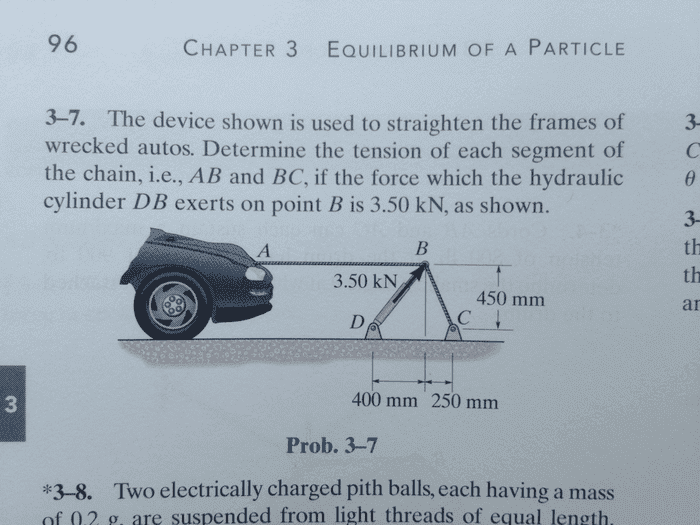# Statics Help (Equilibrium of Forces of Resultant?)

steffyj

## Homework Statement

The device shown is used to straighten the frames of wrecked autos. Determine the tension of each segment of the chain, i.e. AB and BC, if the force which the hydraulic cylinder DB exerts on point B is 3.5kN## Homework Equations

I am trying to figure out if the problem is an equilibrium, or a resultant problem. If it is equilibrium, the equations are:

ƩFx = 0
ƩFy = 0

If it is a resultant:

FDB = TBC - TAB

## The Attempt at a Solution

This was a problem we had in class. I first worked the problem as an equilibrium and got the tensions to be:

Tab = 3.78 kN and Tbc = 2.99 kN, both of which are the right answers.

I then second guessed myself, mainly because I did not understand how a force of 3.5kN could create a tension of 3.78kN, and reworked the problem as a resultant problem. I ended up getting the problem wrong, and although I'm 99.9% that I was truly wrong, I am still confused as to why this problem is treated as an equilibrium problem. It appears to me, that for the device to work, the point B would not be in equilibrium.